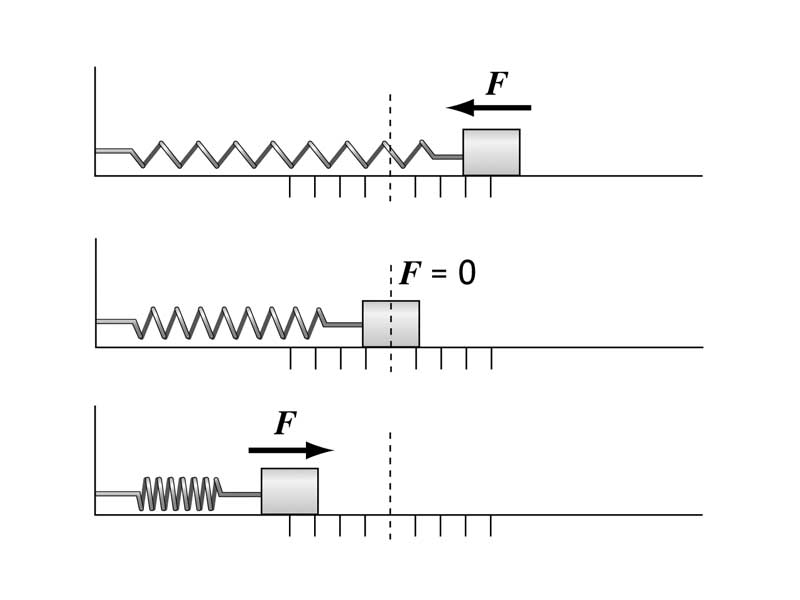#### Simple Harmonic MotionThe mass-spring provides us with a simple model that helps us interpret a range of phenomena involving oscillations and vibrations.

The topic of Simple Harmonic Motion gives us the tools to describe the motion of the frictionless mass-spring and the simple pendulum. Oscillatory motion occurs with these systems because when they are displaced from equilibrium, a force is produced proportional to the displacement from equilibrium. This 'restoring force' acts towards the equilibrium position of the system. However, when the system returns to its equilibrium point, it retains the kinetic energy associated with the work performed by the restoring force, and the mass-spring or the pendulum bob keeps going past equilibrium, which leads to restoring force from the other direction. Each cycle repeats. The mass-spring or pendulum oscillates.

The mass-spring and the simple pendulum are classic problems of Physics 101. There is no escaping them. You must go into the MCAT with a clear understanding of the behavior of these devices. However, the MCAT rarely presents you with the straight-forward model, being more apt to throw you something of a curve ball, such as a compound pendulum or a damped mass-spring. Never panic if the MCAT presents you with a complicated version of a simpler device you have learned. One of the main goals of MCAT preparation is to be prepared to apply fundamental principles within seemingly difficult contexts.

WikiPremed Resources

Harmonic Cards
Chapter from the Wisebridge Learning System for Physics

Simple Harmonic Motion Images
Image gallery for study with links to larger teaching JPEGs for classroom presentation

Question Drill for Harmonic Motion
Conceptual Vocabulary Self-Test

Basic Terms Crossword Puzzle

Basic Puzzle Solution

Learning Goals

ProficiencyBecome comfortably fluent in the language of simple harmonic motion. For example, be able to explain what a 'radian per second' means when talking about a mass-spring.

Understand Hooke's Law in a straightforward way. For example, be able to tell the S.I. units of the spring constant.

Understand the phase relationship of displacement, velocity, and acceleration of a mass-spring.

Be capable of predicting the frequency of a mass-spring or a pendulum based on its intrinsic properties.

Suggested Assignments

Make sure you are familiar with the basic terminology for harmonic motion using the question server. Complete the fundamental terms Crossword Puzzle without pausing. Here is the Solution to the puzzle.

Study the physics cards for harmonic motion.

Read pg. 27 in Examkrackers Physics.

Take a tour of the harmonic motion web resources.

Conceptual Vocabulary for Harmonic Motion

Simple harmonic motion
Simple harmonic motion is the motion of a simple oscillator.
Pendulum
A pendulum is an object that is attached to a pivot point so it can swing freely.
Amplitude
The amplitude is a nonnegative scalar measure of a wave's magnitude of oscillation, the magnitude of the maximum disturbance in the medium during one wave cycle.
Frequency
Frequency is the measurement of the number of occurrences of a repeated event per unit of time.
Spring
A spring is a flexible elastic object used to store mechanical energy usually made out of hardened steel.
Bob
A bob is the weight on the end of a pendulum.
Oscillation
Oscillation is the variation of some measure about a central value (often a point of equilibrium) or between two or more different states.
Harmonic oscillator
A harmonic oscillator is a system which, when displaced from its equilibrium position, experiences a restoring force proportional to the displacement.
Hertz
The hertz is the SI unit of frequency. Its base unit is the cycle per second.
Damping
Damping is any effect that tends to reduce the amplitude of oscillations of an oscillatory system.
Periodic function
A periodic function is a function that repeats its values after some definite period has been added to its independent variable.
Periodicity
Periodicity is the quality of occurring at regular intervals or periods in time or space.
Phase
The phase of an oscillation or wave is the fraction of a cycle corresponding to an offset in the displacement from a specified reference point at time t = 0.
Vibration
Vibration refers to mechanical oscillations about an equilibrium point. The oscillations may be periodic such as the motion of a pendulum or random such as the movement of a tire on a gravel road.
Resonance
Resonance is the tendency of a system to oscillate at maximum amplitude at a certain frequency.
Small-angle approximation
The small-angle approximation is a useful simplification of the laws of trigonometry which is only approximately true for finite angles, but correct in the limit as the angle approaches zero.
Isochronous
Isochronous means having an equal time difference or occurring simultaneously.
Double pendulum
A double pendulum is a system of two simple pendulums on a common mounting which move in anti-phase.The WikiPremed MCAT Course is a comprehensive course in the undergraduate level general sciences. Undergraduate level physics, chemistry, organic chemistry and biology are presented by this course as a unified whole within a spiraling curriculum. Please read our policies on Privacy and Shipping & Returns.  Contact Us. MCAT is a registered trademark of the Association of American Medical Colleges, which does not endorse the WikiPremed Course. WikiPremed offers the customers of our publications or our teaching services no guarantees regarding eventual performance on the MCAT.WikiPremed is a trademark of Wisebridge Learning Systems LLC. The work of WikiPremed is published under a Creative Commons Attribution NonCommercial ShareAlike License. There are elements of work here, such as a subset of the images in the archive from WikiPedia, that originated as GNU General Public License works, so take care to follow the unique stipulations of that license in printed reproductions.